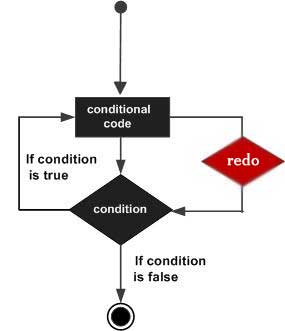# Perl redo 语句

Perl redo 语句
Perl 循环

Perl redo 语句直接转到循环体的第一行开始重复执行本次循环，redo语句之后的语句不再执行，continue语句块也不再执行。

continue 语句可用在 while 和 foreach 循环中。

redo [LABEL]#/usr/bin/perl

$a = 0; while($a < 10){
if( $a == 5 ){$a = $a + 1; redo; } print "a =$a\n";
}continue{
$a =$a + 1;
}


a = 0
a = 1
a = 2
a = 3
a = 4
a = 6
a = 7
a = 8
a = 9


Perl 循环

• 回顶